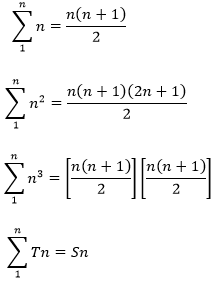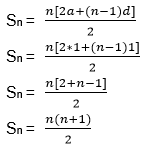# Sequence and Series Introduction

A sequence is defined as the succession of numbers formed according to some definite rules. A clear introduction of sequence and series is given in this article.

A sequence is a group of numbers in an ordered form which obeys a certain pattern. The sequence and series can be finite or infinite depending upon the number of terms in as finite or infinite respectively. A series can be constructed by adding or subtracting the terms of a sequence.  The nth term is denoted by  an or Tn and is a general term of sequence/series.

A finite sequence is a sequence which has a finite number of terms. For example {2,4,6,8} is a sequence of multiples of 2 less than 9.

An infinite sequence has an unlimited number of terms. For example 3,6,9,…

Types of Sequence and Series

There are three types of the sequence as mentioned below:

· Arithmetic Sequence

· Geometric Sequence

· Fibonacci Sequence

And, we deal with two types of series mainly.

· Arithmetic Series

· Geometric Series

Arithmetic Progression

A sequence of the numbers is called an arithmetic progression when there is a common difference between two consecutive terms.

Important Formulas

nth term of A.P = a + (n – 1) d

(n+1)th term = a + nd

Sum of n terms of an A.P = $\frac{n[2a +(n-1)d]}{2}$ where a is the first term and d is the common difference.

Properties of Arithmetic sequences

1) The resulting sequence of an AP remains the same if a constant is added or subtracted from each term of an A.P.

2) If we select terms in the regular interval from an A.P, these selected terms will also be in AP.

3) The resulting sequence of an AP remains the same if a constant non-zero number divided or multiplied by each term of an A.P.

4) Selection of terms in A.P.

3 terms: a – d, a, a + d

4 terms: a – 3d, a – d, a + d, a + 3d

5 terms: a – 2d, a – d, a, a + d, a + 2d

## Geometric Progression

A sequence of the numbers is a geometric progression when there is a common ratio that comes by multiplying or dividing the two consecutive terms.

General Form: a, ar, ar2, ar3, …, arn

nth term of a G.P = arn-1

Sum of n terms of a G.P = a(1-rn)/(1-r)

where r is the common ratio and a is the first term.

### Fibonacci Sequence

A sequence of the numbers is called a Fibonacci sequence when the required term is obtained by adding the two terms before that.

 The formula of the Fibonacci Sequence is an = an – 2 + an – 1, Where n > 2 Also known as the Recursive Formula.

## What is a series in math?

The summation of all the terms of the sequence is called Series. It is denoted as Sn. For example, If we are given the sequence 2, 4, 6, 8, ….Then the series is 2+4+6+8+ ….

 Arithmetic Series Geometric Series General Form {a + (a + d) + (a + 2d) + (a + 3d) + ………} $\sum_{n=1}^{\infty} ar^n = a + ar + ar^2 + ….ar^n$ nth term an = a + (n – 1) d an = a rn – 1 Sum of n terms $\frac{n[2a +(n-1)d]}{2}$ $S_n= \frac{a(1-r^n}{1-r}$

Important summations:## Solved Problems for IIT JEE

Problem 1: Find the nth term of the given series

1, (1 + 2), (1 + 2 + 3), (1 + 2 + 3 + 4), . . . . . . . . . . . . . .

Solution: Here nth term = (1 + 2 + 3 + 4 + 5 . . . . . . . . . . . .+ n)

This is an arithmetic series with First term = 1 and Common difference = 1

Then,Which is the nth term of the given series.

Problem 2: If the 9th term of an A.P. is zero, then find the ratio of its 29th term and 19th term.

Solution:

9th term = a + (9 – 1) d = 0

=> a + 8d = 0

or a = -8d

Now,

(29th term)/(19th term) = (a + 28d) / (a + 18d) = ((-8d + 28d)) / ((-8d + 18d)) = 20d / 10d = 2

Problem 3: If the nth term of an A.P. is (2n-1) then find the sum of its n terms.

Solution: We know, $\sum_1^n T_n = S_n$ $\sum_1^n T_n = \sum_1^n (2n-1) = 2 \sum_1^n n – \sum_1^n 1$

= 2 (n (n + 1)) / 2 – n

= n2

Problem 4: If the nth term of the series 63, 65, 67, 69 . . . . . . . . . . . . and mth term of the series 3, 10, 17, 24 . . . . . . . . . . . . . . . . is equal then find the relation between m and n.

Solution: The nth term of the first series = 63 + (n – 1)2 = 61 + 2n

The mth term of the second series = 3 + (m – 1)7 = 7m – 4

If both are equal, 61 + 2n = 7m – 4

or 7m – 2n = 65

Problem 5: If a, b, c are in A.P. then find the value of (a-c)2 / (b2-ac).

Solution: Three terms in an A.P. can be taken as

A – D, A, A + D

So here,

a = A – D

b = A

c = A + D

a – c = -2D

Now,

b2 = A2

ac = A2 – D2

b2 – ac = D2

So, (a – c)2/(b– ac) = (4D2) / D2 = 4

Problem 6: Find the sum of the first 20 terms of the sequence 0.7, 0.77, 0.777 . . . . . . . . upto 20 terms.

Solution:

$0.7+0.77+0.777+……\text \ upto \ 20 \text \ terms\\ \Rightarrow 7(0.1+0.11+0.1111+……..\text \ upto \ 20 \text \ terms)\\ \Rightarrow 7(\frac{1}{10}+\frac{11}{10^{2}}+\frac{111}{10^{3}}+……\text \ upto \ 20 \text \ terms)\\ \Rightarrow \frac{7}{9}(\frac{9}{10}+\frac{99}{10^{2}}+\frac{999}{10^{3}}+……\text \ upto \ 20 \text \ terms)\\ \Rightarrow \frac{7}{9}((1-\frac{1}{10})+(1-\frac{1}{100})+(1-\frac{1}{1000})+……\text \ upto \ 20 \text \ terms)\\ \Rightarrow \frac{7}{9}(20-(\frac{1}{10})+(\frac{1}{100})+(\frac{1}{1000})+……\text \ upto \ 20 \text \ terms)\\ \Rightarrow \frac{7}{9}(20-(\frac{1}{10})(\frac{1-(\frac{1}{10})^{20})}{1-\frac{1}{10}}))\\ \Rightarrow \frac{7}{9}(20-(\frac{1}{9})(1-(\frac{1}{10})^{20}))\\ \Rightarrow \frac{7}{81}(179+(\frac{1}{10})^{20})\\$

Problem 7: If $(10)^{9}+2(11)^{1}(10)^{8}+3(11)^{2}{10}^{7}+…..+10(11)^{9}=k(10)^{9},$ then find the value of k.

Solution:

$(10)^{9}+2(11)^{1}(10)^{8}+3(11)^{2}{10}^{7}+…..+10(11)^{9}=k(10)^{9}\\ \text \ Dividing \ by \ (10)^{9} \ on \ both \ sides \\ 1+2(\frac{11}{10}+3((\frac{11}{10})^{2})+…….10((\frac{11}{10})^{9})=k\rightarrow (1)\\ \text \ Multiplying \ \frac{11}{10}\ on \ both \ side \ of \ the \ above \ equation \ we \ get\\ (\frac{11}{10}+2((\frac{11}{10})^{2})+3((\frac{11}{10})^{3})…….10((\frac{11}{10})^{10})=(\frac{11}{10})^{10})k\rightarrow (2)\\ \text \ Subtracting \ (2) \ from \ (1) \ we \ get \\ k-\frac{11}{10}k=1+\frac{11}{10}+(\frac{11}{10})^{2})+(\frac{11}{10})^{3})+………..+(\frac{11}{10})^{9})-10(\frac{11}{10})^{10})\\ \Rightarrow k(1-\frac{11}{10})=\frac{1(\frac{11}{10})^{10})-1}{(\frac{11}{10})-1}-10(\frac{11}{10})^{10})\\ \Rightarrow k(1-\frac{11}{10})=-10\\ \Rightarrow k=100\\$

Problem 8: Let $a_{1},a_{2},a_{3}…..$ be a harmonic progression with its first term as 5 and 20th term as 25. The least positive integer n for which $a_{n}<0$ is _____.

Solution:

If $a_{1},a_{2},a_{3}…..$ be a harmonic progression [HP], then $\frac{1}{a_{1}},\frac{1}{a_{2}},\frac{1}{a_{3}}…..$ will be in arithmetic progression [AP].

First-term of AP, $\frac{1}{a_{1}}=\frac{1}{5}$

Term 20 of AP,

$\frac{1}{a_{20}}=\frac{1}{25}\\\Rightarrow \frac{1}{5}+19d=\frac{1}{25}\\ \Rightarrow d=\frac{-4}{19*25}\\$

We have to find the least positive integer n for which $a_{n}<0\\$ $\Rightarrow \frac{1}{5}+(n-1)d<0\\ \Rightarrow \frac{1}{5}+(n-1)(\frac{-4}{19*25})<0\\ \Rightarrow (n-1)>\frac{95}{4}\\ \Rightarrow n>24.75\\$

Problem 9: Find the sum of all natural numbers lying between 101 and 199 which are multiples of 5.

Solution:

We know the nearest number to 101 which is a multiple of 5 and lies between 101 and 199 is 105. Similarly 195 is the nearest number to 199 which is a multiple of 5 which lies between 101 and 199. So we take the last term of A.P as 195.

We have first term, a = 105

Common difference, d = 5

Last term, l = 195

l = a+(n-1) d .

195 = 105+(n-1)5

195-105 = ( n-1)5

5n-5 = 90

5n = 90+5 = 95

n = 95/5 = 19

Sum of n terms = n/2(a+l)

= 19/2(105+195) = 2850

Hence the required sum is 2850.

### Also Check:

Arithmetic progression solved examples

Geometric progression solved examples